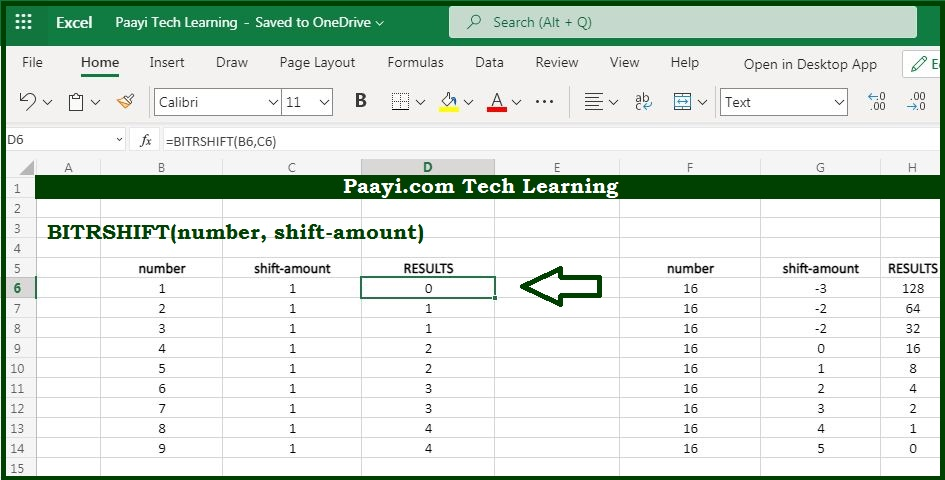# Learn How to Use Microsoft Excel BITRSHIFT Function

Written by | 0 Comments | 511 Views

In this article, you will learn how to use the Microsoft Excel BITRSHIFT function and its prime function in Microsoft Excel. You will also get to know the Microsoft Excel BITRSHIFT function return value and syntax with the help of some examples.

### Microsoft Excel BITRSHIFT Function

The main function of the Microsoft Excel BITRSHIFT function is used to return a number shifted right by some number of bits. So with the help of the BITRSHIFT function, you can able to shift a number by the specified number of bits. Also, you can effectively half or double the number specified number of times.

### Return Value of BITRSHIFT Function

The return value will be the decimal number.

### Syntax of BITRSHIFT Function

=BITRSHIFT(number, shift_amount)

Where the argument:

number: It is the number to be bit shifted.

shift_amount: It is the amount of bits to be shifted right, in case negative it shifts the bits to the left instead.

## How to Use Microsoft Excel BITRSHIFT Function?The shift_amount can be negative or positive and if a negative number is provided, the bits are shifted to the left. For any bit shift that results in an integer overflow, where the result is greater than 2^48-1, the BITLSHIFT function will return the #NUM! error.